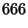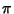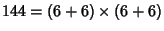## Beast Number

The occult number of the beast'' associated in the Bible with the Antichrist. It has figured in many numerological studies. It is mentioned in Revelation 13:18: Here is wisdom. Let him that hath understanding count the number of the beast: for it is the number of a man; and his number is 666.''

The beast number has several interesting properties which numerologists may find particularly interesting (Keith 1982-83). In particular, the beast number is equal to the sum of the squares of the first 7 Primes(1)

satisfies the identity(2)

whereis the Totient Function, as well as the sum(3)

The number 666 is a sum and difference of the first three 6th Powers,(4)

(Keith). Another curious identity is that there are exactly two ways to insert +'' signs into the sequence 123456789 to make the sum 666, and exactly one way for the sequence 987654321,(5)(6)

(Keith). 666 is a Repdigit, and is also a Triangular Number(7)

In fact, it is the largest Repdigit Triangular Number (Bellew and Weger 1975-76). 666 is also a Smith Number. The first 144 Digits of, whereis Pi, add to 666. In addition(Blatner 1997).

A number of the formwhich contains the digits of the beast number 666'' is called an Apocalyptic Number, and a number having 666 digits is called an Apocalypse Number.

References

Bellew, D. W. and Weger, R. C. Repdigit Triangular Numbers.'' J. Recr. Math. 8, 96-97, 1975-76.

Blatner, D. The Joy of Pi. New York: Walker, back jacket, 1997.

Castellanos, D. The Ubiquitous.'' Math. Mag. 61, 153-154, 1988.

Hardy, G. H. A Mathematician's Apology, reprinted with a foreword by C. P. Snow. New York: Cambridge University Press, p. 96, 1993.

Keith, M. The Number of the Beast.'' http://users.aol.com/s6sj7gt/mike666.htm.

Keith, M. The Number 666.'' J. Recr. Math. 15, 85-87, 1982-1983.